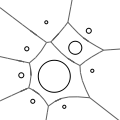CGAL 4.12.1 - 2D Apollonius Graphs (Delaunay Graphs of Disks)
2D Apollonius Graphs (Delaunay Graphs of Disks) ReferenceMenelaos Karavelas and Mariette Yvinec
Algorithms for computing the Apollonius graph in two dimensions. The Apollonius graph is the dual of the Apollonius diagram, also known as the additively weighted Voronoi diagram. The latter can be thought of as the Voronoi diagram of a set of disks under the Euclidean metric, and it is a generalization of the standard Voronoi diagram for points. The algorithms provided are dynamic.

Introduced in: CGAL 3.0
Depends on: 2D Triangulation Data Structure
BibTeX: cgal:ky-ag2-18b
Windows Demo: 2D Apollonius Graph
Common Demo Dlls: dlls

An Apollonius graph is the dual of the Apollonius diagram, also known as the additively weighted Voronoi diagram. It is essentially the Voronoi diagram of a set of disks, where the distance of a point of the plane from a disk is defined as the Euclidean distance of the point and the center of the circle, minus the radius of the disk.

CGAL provides the class CGAL::Apollonius_graph_2<Gt,Agds> for computing the 2D Apollonius graph. The two template parameters must be models of the ApolloniusGraphTraits_2 and ApolloniusGraphDataStructure_2 concepts. The first concept is related to the geometric objects and predicates associated with Apollonius graphs, whereas the second concept refers to the data structure used to represent the Apollonius graph. The classes Apollonius_graph_traits_2<K,Method_tag> and Triangulation_data_structure_2<Vb,Fb> are models of the aforementioned concepts.

## Concepts

• ApolloniusSite_2
• ApolloniusGraphDataStructure_2
• ApolloniusGraphVertexBase_2
• ApolloniusGraphTraits_2
• ApolloniusGraphHierarchyVertexBase_2

## Classes

• CGAL::Apollonius_graph_2<Gt,Agds>
• CGAL::Apollonius_site_2<K>
• CGAL::Apollonius_graph_vertex_base_2<Gt,StoreHidden>
• CGAL::Apollonius_graph_traits_2<K,Method_tag>
• CGAL::Apollonius_graph_filtered_traits_2<CK,CM,EK,EM,FK,FM>
• CGAL::Apollonius_graph_hierarchy_2<Gt,Agds>
• CGAL::Apollonius_graph_hierarchy_vertex_base_2<Agvb>

Concepts

## Classes

class  CGAL::Apollonius_graph_2< Gt, Agds >
The class Apollonius_graph_2 represents the Apollonius graph. More...

class  CGAL::Apollonius_graph_filtered_traits_2< CK, CM, EK, EM, FK, FM >
The class Apollonius_graph_filtered_traits_2 provides a model for the ApolloniusGraphTraits_2 concept. More...

class  CGAL::Apollonius_graph_hierarchy_2< Gt, Agds >
We provide an alternative to the class Apollonius_graph_2<Gt,Agds> for the dynamic construction of the Apollonius graph. More...

class  CGAL::Apollonius_graph_hierarchy_vertex_base_2< Agvb >
The class Apollonius_graph_hierarchy_vertex_base_2 provides a model for the ApolloniusGraphHierarchyVertexBase_2 concept, which is the vertex base required by the Apollonius_graph_hierarchy_2<Gt,Agds> class. More...

class  CGAL::Apollonius_graph_traits_2< K, Method_tag >
The class Apollonius_graph_traits_2 provides a model for the ApolloniusGraphTraits_2 concept. More...

class  CGAL::Apollonius_graph_vertex_base_2< Gt, StoreHidden >
The class Apollonius_graph_vertex_base_2 provides a model for the ApolloniusGraphVertexBase_2 concept which is the vertex base required by the ApolloniusGraphDataStructure_2 concept. More...

class  CGAL::Apollonius_site_2< K >
The class Apollonius_site_2 is a model for the concept ApolloniusSite_2. More...